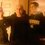# Solve for equal sides of isosceles triangle given base and two perpendiculars from base to the two sides

I cannot find the specific problem but it summarized as follows:

Isosceles triangle $ABC$ with $AB=AC$ and $BC=60$.
A point $D$ on base $BC$ is located such that a perpendicular to side $AB$, denoted as $DE$, has length 16 and a perpendicular to side $AC$, denoted as $DF$, has length 32. Points $E$ and $F$ are on $AB$ and $AC$ respectively. What is the length of the two equal sides of triangle $ABC$?

The correct answer shown was 50. I got a different answer using similar triangles etc. I checked and rechecked and continued to get my original answer. I then constructed the posted answer graphically and while the one perpendicular was equal to 16, it resulted in the other perpendicular being ~38.75.

In my answer, each of the two equal sides were ~43.148. Moreover, when I graphed the problem, the two perpendiculars measured 16 and 32 respectively.

At this point, I am wondering if my rendering of the image is wrong as no drawing was included in the problem.Note by Greg Grapsas
5 years, 5 months ago

This discussion board is a place to discuss our Daily Challenges and the math and science related to those challenges. Explanations are more than just a solution — they should explain the steps and thinking strategies that you used to obtain the solution. Comments should further the discussion of math and science.

When posting on Brilliant:

• Use the emojis to react to an explanation, whether you're congratulating a job well done , or just really confused .
• Ask specific questions about the challenge or the steps in somebody's explanation. Well-posed questions can add a lot to the discussion, but posting "I don't understand!" doesn't help anyone.
• Try to contribute something new to the discussion, whether it is an extension, generalization or other idea related to the challenge.

MarkdownAppears as
*italics* or _italics_ italics
**bold** or __bold__ bold
- bulleted- list
• bulleted
• list
1. numbered2. list
1. numbered
2. list
Note: you must add a full line of space before and after lists for them to show up correctly
paragraph 1paragraph 2

paragraph 1

paragraph 2

[example link](https://brilliant.org)example link
> This is a quote
This is a quote
    # I indented these lines
# 4 spaces, and now they show
# up as a code block.

print "hello world"
# I indented these lines
# 4 spaces, and now they show
# up as a code block.

print "hello world"
MathAppears as
Remember to wrap math in $$ ... $$ or $ ... $ to ensure proper formatting.
2 \times 3 $2 \times 3$
2^{34} $2^{34}$
a_{i-1} $a_{i-1}$
\frac{2}{3} $\frac{2}{3}$
\sqrt{2} $\sqrt{2}$
\sum_{i=1}^3 $\sum_{i=1}^3$
\sin \theta $\sin \theta$
\boxed{123} $\boxed{123}$

Sort by:

Let point $G$ be foot of perpendicular from A on $BC$. $\triangle EBD$ is similar to $\triangle DFC$ and to $\triangle BGA$. $BD=20, BE=12, AG=40, AB=50$

It looks like the answer is in fact 50.

- 5 years, 5 months ago

Can you include a drawing? If point D is on the base then BE cant be 12 sonce it the hypotenuse of triangle EDB

- 5 years, 5 months ago

- 5 years, 5 months ago

Thank you...when i saw it i realized my stupid mistake...i had df correct but i foolishly had DE perpendicular to the base instead of BA and just couldnt see it until you sent me the drawing

- 5 years, 5 months ago